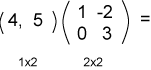No. The dimensions are not correct.

# Row Matrix always on the Left

When a row matrix is multiplied by a rectangular matrix, the row matrix must be on the left.

Look at the dimensions of the operands and of the result:

```Row Matrix  x  Matrix   =   Result Row Matrix

1 x M        M x C           1 x C
```

A 1xC matrix is a row matrix of "C" number of columns. For each row in the following table, decide if the product can be formed and what the dimensions of the result will be.

First Operand Second Operand Result
3 x 3 3 x 1
1 x 2 2 x 2
1 x 2 2 x 3
4 x 4 3 x 1
1 x 5 5 x 3

### QUESTION 11:

Perform the following multiplication: